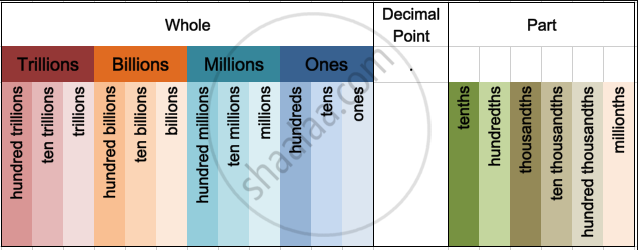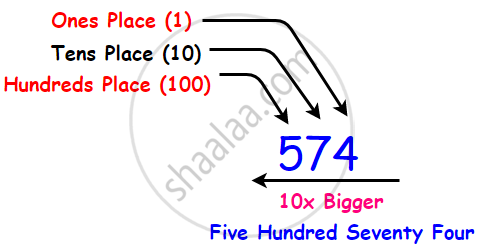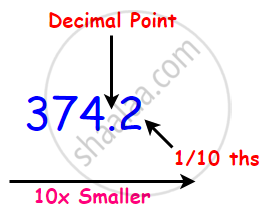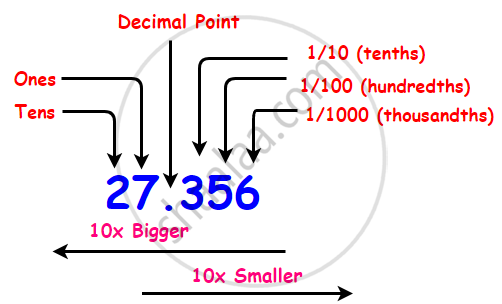# Place Value in the Context of Decimal Fraction

## Definition

Place value: Place value can be defined as the value represented by a digit in a number on the basis of its position in the number.

## Notes

### Place Value:#### 1. Place value of the whole number:

• In maths, every digit in a number has a place value.

• Place value can be defined as the value represented by a digit in a number on the basis of its position in the number.• In 574, 5 is in hundred's place and its place value is 500,
7 is in ten's place and its place value is 70,
4 is in one's place and its place value is 4.

#### 2. Place value of a decimal number:

Decimal numbers are fractions or mixed numbers with denominators of powers of ten.In a decimal number, the digits to the left of the decimal point represent a whole number. The digits to the right of the decimal represent the parts. The place value of the digits becomes 10 times smaller.

The first digit on the right of the decimal point means tenths i.e. 1/10ths.In 27.356,
27 is the whole number part,
2 is in ten's place and its place value is 20,
7 is in one's place, and its place value is 7.

There are three digits to the right of the decimal point,
3 is in the tenth's place, and its place value is 0.3 or 3/10
5 is in the hundredth's place, and its place value is 0.05 or 5/100
6 is in the thousandth's place, and its place value is 0.006 or 6/1000.

## Example

Write the following decimals in the place value table.
0.4

 Hundreds Tens Ones Tenths Hundredths Thousandths 0 0 0 4 0 0

## Example

Write the following decimals in the place value table.
0.467

 Hundreds Tens Ones Tenths Hundredths Thousandths 0 0 0 4 6 7

## Example

Write the following decimals in the place value table.
10.408

 Hundreds Tens Ones Tenths Hundredths Thousandths 1 0 4 0 8

## Example

Given the place value table, write the number in decimal form.

 Hundreds Tens Ones Tenths Hundredths Thousandths 0 0 2 5 7 0

2 × 10 + 5 × 1 + 7/10

= 20 + 5 + 7/10

= 25 + 7/10

= 25 + 0.7

= 25.7

## Example

Given the place value table, write the number in decimal form.

 Thousands Hundreds Tens Ones Tenths Hundredths Thousandths 0 1 9 7 6 8 0

1 × 100 + 9 × 10 + 7 × 1 + 6/10 + 8/100

⇒ 100 + 90 + 7 + 6/10 + 8/100

⇒ 197 + (60 + 8)/100

⇒ 197 + 68/100

⇒ 197 68/100

⇒ 197.68

## Example

Given the place value table, write the number in decimal form.

 Thousands Hundreds Tens Ones Tenths Hundredths Thousandths 7 3 2 1 0 8 9

7 × 1000 + 3 × 100 + 2 × 10 + 1 × 0 + 8/100 + 1/1000.

= 7000 + 300 + 20 + 1 + 0 + 8/100 + 1/1000.

= 7321 + (80 + 9)/1000

= 7321 + 89/1000

= 7321 89/1000

= 7321.089

If you would like to contribute notes or other learning material, please submit them using the button below.

### Shaalaa.com

How To Write Numbers In The Place Value Table? [00:04:51]
S
0%